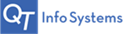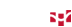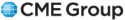Overview
• Overview
• Corn - CBOT
Month
Last Change High Low Open Volume Time OptionChart
Sep '19 425^4  S +3^2 426^4 418^2 423^0 118411 1:19:59 pm Option Chart
Dec '19 431^4  S +4^6 432^6 424^0 428^0 171773 1:19:59 pm Option Chart
Mar '20 440^4  S +4^6 441^2 433^0 437^0 42977 1:19:59 pm Option Chart
May '20 444^2  S +4^0 445^2 437^2 441^2 9728 1:19:59 pm Option Chart
Jul '20 447^2  S +3^4 448^2 441^0 444^4 9199 1:19:55 pm Option Chart
Hard Red Winter Wheat - KCBT
Month
Last Change High Low Open Volume Time OptionChart
Sep '19 431^4  S +2^2 435^4 426^4 432^6 28433 1:19:49 pm Option Chart
Dec '19 449^2  S +0^6 453^6 445^4 447^4 13906 9:28:36 am Option Chart
Mar '20 467^2  S -0^4 472^0 464^2 466^4 5239 9:25:26 am Option Chart
May '20 479^0  S -1^0 484^0 477^0 482^2 931 11:24:48 am Option Chart
Jul '20 484^6  S -1^0 489^2 483^0 487^6 752 10:00:01 am Option Chart
Wheat - CBOT
Month
Last Change High Low Open Volume Time OptionChart
Sep '19 487^2  S 0^0 491^6 483^2 490^0 55578 1:19:57 pm Option Chart
Dec '19 498^2  S -0^6 505^0 495^0 499^0 25482 1:19:51 pm Option Chart
Mar '20 510^6  S -0^6 517^2 507^6 512^6 12853 1:19:45 pm Option Chart
May '20 517^2  S -0^6 523^2 514^2 519^0 1920 1:14:52 pm Option Chart
Jul '20 519^6  S -0^6 525^4 517^0 522^2 1365 1:14:57 pm Option Chart
All quotes are delayed by at least 10 minutes.

Powered By:Quote Data Provided by